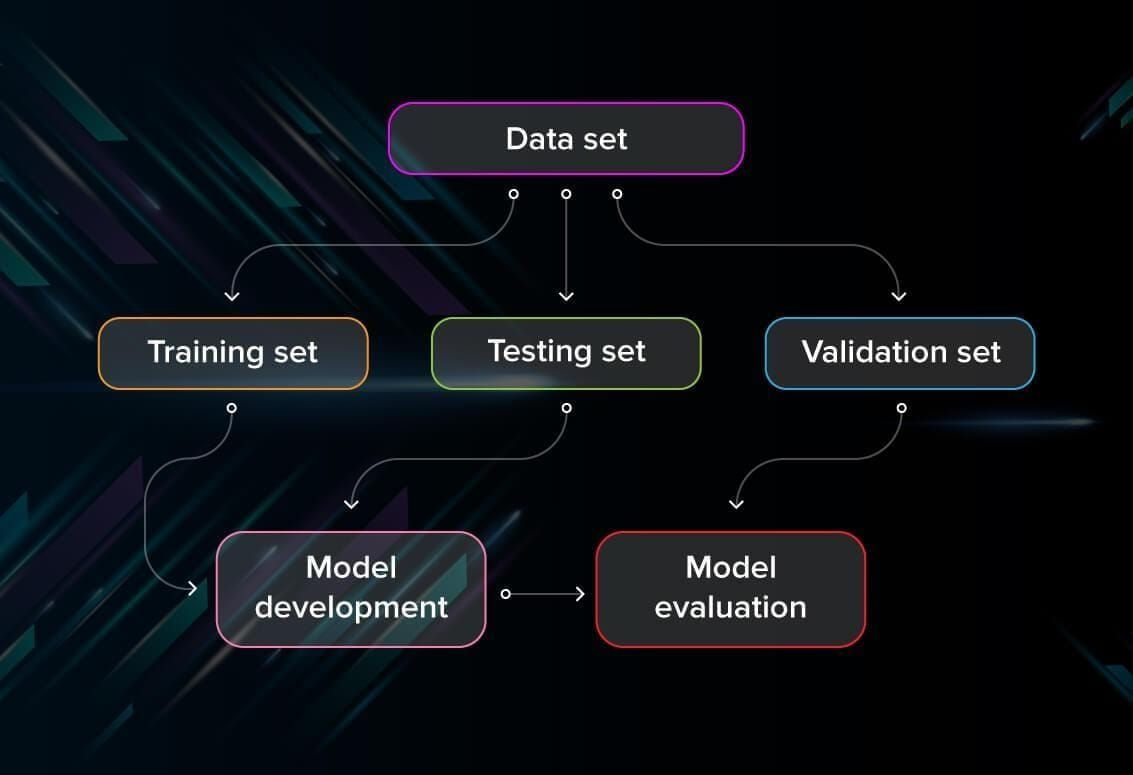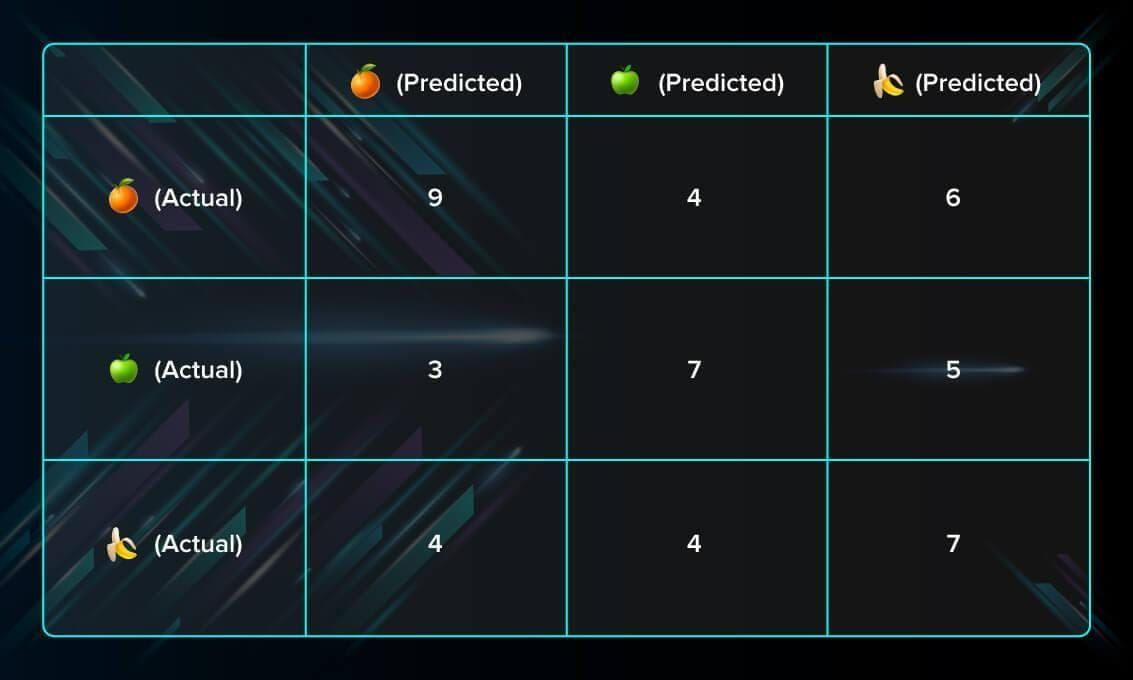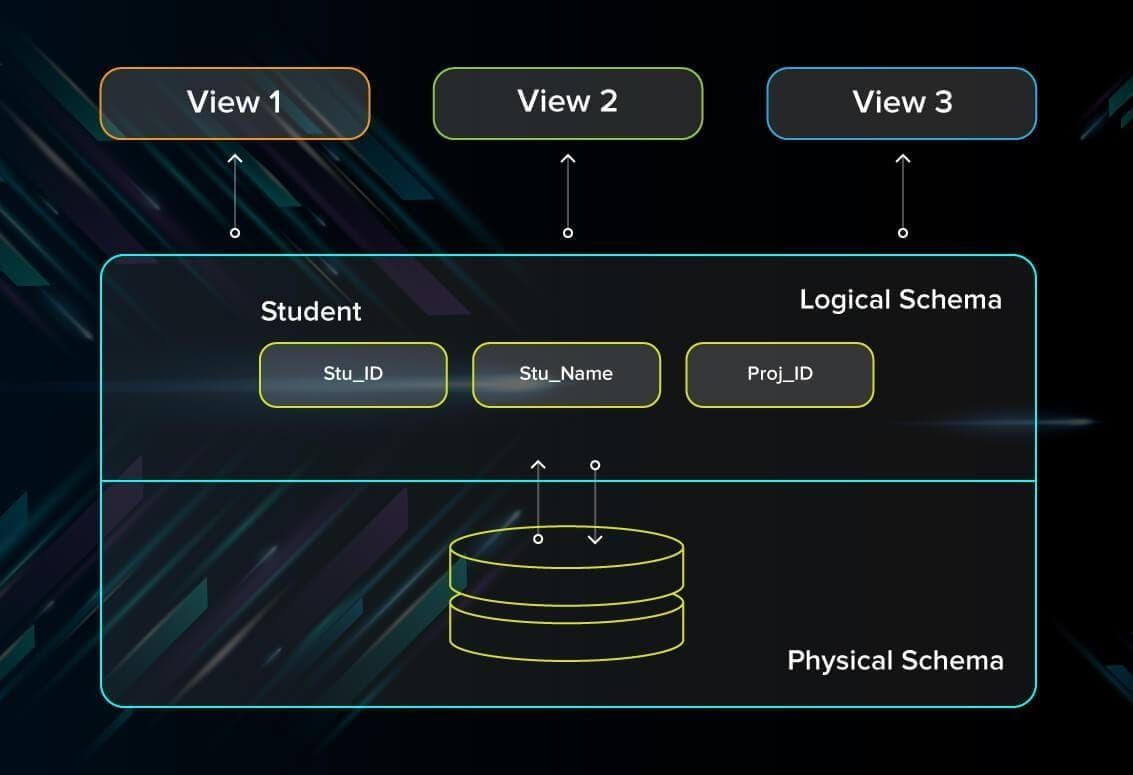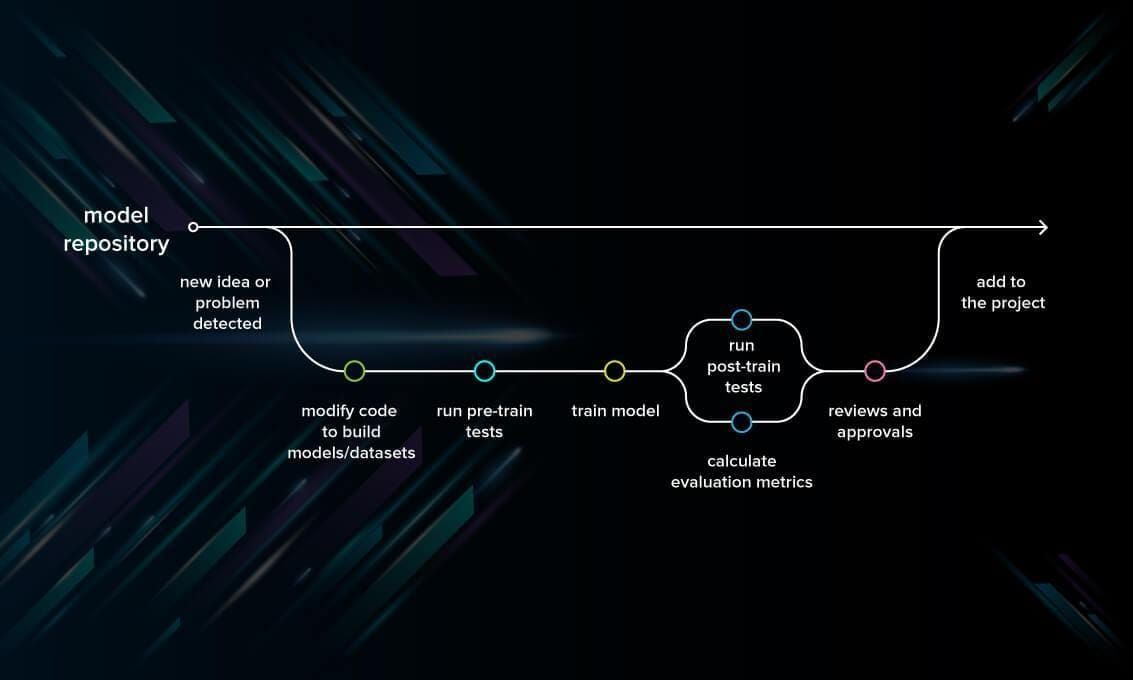# Machine Learning Testing: A Step to Perfection

In this post, we’ll discuss strategies for effective ML testing and share some practical tips from our experience as an ML project outsourcing team. You will learn how to test and evaluate models, overcome common bottlenecks, and more.

## What is the goal of ML testing?

First of all, what are we trying to achieve when performing ML testing, as well as any software testing whatsoever?

• Quality assurance is required to make sure that the software system works according to the requirements. Were all the features implemented as agreed? Does the program behave as expected? All the parameters that you test the program against should be stated in the technical specification document.
• Moreover, software testing has the power to point out all the defects and flaws during development. You don’t want your clients to encounter bugs after the software is released and come to you waving their fists. Different kinds of testing allow us to catch bugs that are visible only during runtime.

However, in machine learning, a programmer usually inputs the data and the desired behavior, and the logic is elaborated by the machine. This is especially true for deep learning. Therefore, the purpose of machine learning testing is, first of all, to ensure that this learned logic will remain consistent, no matter how many times we call the program.

## Model evaluation in machine learning testing

Usually, software testing includes:

• Unit tests. The program is broken down into blocks, and each element (unit) is tested separately.
• Regression tests. They cover already tested software to see if it doesn’t suddenly break.
• Integration tests. This type of testing observes how multiple components of the program work together.

Moreover, there are certain rules that people follow: don’t merge the code before it passes all the tests, always test newly introduced blocks of code, when fixing bugs, write a test that captures the bug.

Machine learning adds up more actions to your to-do list. You still need to follow ML’s best practices. Moreover, every ML model needs not only to be tested but evaluated. Your model should generalize well. This is not what we usually understand by testing, but evaluation is needed to make sure that the performance is satisfactory.First of all, you split the database into three non-overlapping sets. You use a training set to train the model. Then, to evaluate the performance of the model, you use two sets of data:

• Validation set. Having only a training set and a testing set is not enough if you do many rounds of hyperparameter-tuning (which is always). And that can result in overfitting. To avoid that, you can select a small validation data set to evaluate a model. Only after you get maximum accuracy on the validation set, you make the testing set come into the game.
• Test set (or holdout set). Your model might fit the training dataset perfectly well. But where are the guarantees that it will do equally well in real-life? In order to assure that, you select samples for a testing set from your training set — examples that the machine hasn’t seen before. It is important to remain unbiased during selection and draw samples at random. Also, you should not use the same set many times to avoid training on your test data. Your test set should be large enough to provide statistically meaningful results and be representative of the data set as a whole.But just as test sets, validation sets “wear out” when used repeatedly. The more times you use the same data to make decisions about hyperparameter settings or other model improvements, the less confident you are that the model will generalize well on new, unseen data. So it is a good idea to collect more data to ‘freshen up’ the test set and validation set.

### Cross-validation

Cross-validation is a model evaluation technique that can be performed even on a limited dataset. The training set is divided into small subsets, and the model is trained and validated on each of these samples.

#### k-fold cross-validation

The most common cross-validation method is called k-fold cross-validation. To use it, you need to divide the dataset into $k$ subsets (also called folds) and use them $k$ times. For example, by breaking the dataset into 10 subsets, you will perform a 10-fold cross-validation. Each subset must be used as the validation set at least once.This method is useful to test the skill of the machine learning model on unseen data. It is so popular because it is simple to apply, works well even with relatively small datasets, and the results you get are generally quite accurate. If you want to learn more about how to cross-validate the model, check out a more detailed explanation on Medium.

#### Leave-one-out cross-validation

In this method, we train the model on all the data samples in the set except for one data point that is used to test the model. By repeating this process iteratively, each time leaving a different data point as a testing set, you get to test the performance for all the data.

The benefit of the method is low bias since all the data points are used. However, it also leads to higher variation in testing because we are testing the model against just one data point each time.

Cross-validation provides for more efficient use of the data and helps to better assess the accuracy of the model.

### Evaluate models using metrics

Evaluating the performance of the model using different metrics is integral to every data science project. Here is what you have to keep an eye on:

#### Accuracy

Accuracy is a metric for how much of the predictions the model makes are true. The higher the accuracy is, the better. However, it is not the only important metric when you estimate the performance.

$$Accuracy \equiv \frac{True\ Positives + True\ Negatives}{True\ Positives + False\ Positives + True\ Negatives + False\ Negatives}$$

#### Loss

Loss describes the percentage of bad predictions. If the model’s prediction is perfect, the loss is zero; otherwise, the loss is greater.

#### Precision

The precision metric marks how often the model is correct when identifying positive results. For example, how often the model diagnoses cancer to patients who really have cancer.

$$Precision \equiv \frac{True\ Positives}{True\ Positives + False\ Positives}$$

#### Recall

This metric measures the number of correct predictions, divided by the number of results that should have been predicted correctly. It refers to the percentage of total relevant results correctly classified by your algorithm.

$$Recall \equiv \frac{True\ Positives}{True\ Positives + False\ Negatives}$$

#### Confusion matrix

A confusion matrix is an $N\times N$ square table, where $N$ is the number of classes that the model needs to classify. Usually, this method is applied to classification where each column represents a label. For example, if you need to categorize fruits into three categories: oranges, apples, and bananas, you draw a $3\times3$ table. One axis will be the actual label, and the other will be the predicted one.## Best practices for ML model debugging

Having evaluated the performance, we still have to figure out where and why the errors occur.

ML debugging is a bit different from debugging any other software system. Poor quality of predictions made by an ML model does not necessarily mean there is a bug. You have to investigate a broader range of causes than you would in traditional programming: maybe it is the data that contains errors or hyperparameters are not adjusted well. This makes debugging ML models quite challenging.

## Data debugging

First of all, you should start with data debugging because the accuracy of predictions made by the model depends not only on the algorithm but on the quality of data itself.

### Database schema

One tool that helps you to check whether the data contains the expected statistical values is the data schema.

A database schema is like a map that describes the logic of the database: how the data is organized and what the relationship between the samples is. It may include certain rules like:

• Ensure that the submitted values are within the 1-5 range (in ratings, for example).
• Check that all the images are in the JPEG format.

The scheme can be of two types:

• Physical. It describes how the data will be stored, its formats, etc.
• Logical. This type represents the logical components of the database in the form of tables, tags, or schemes.The engineered data should be checked separately. While raw data might be okay, engineered data went through some changes and can look totally different. For example, you can write tests checking that the outliers are handled or that the missing values were replaced by mean or default values.

## Model debugging

Once you have tested your data, you can proceed with model debugging.

### Establish a baseline

When you set a baseline and compare your model against it, you quickly test the model’s quality. Establishing a baseline means that you use a simple heuristic to predict the label. If your trained model performs worse than its baseline, you need to improve your model. For example, if your model solves a classification problem, the baseline is predicting the most common label.

Once you validate your model and update it, you can use it as a baseline for newer versions of the model. An updated, more complex model must perform better than a less complex baseline.

### Write ML unit tests

This kind of ML testing is more similar to traditional testing: you write and run tests checking the performance of the program. Applying the tests, you catch bugs in different components of the ML program. For example, you can test that the hidden layers in a neural network are configured correctly. If you are interested in diving deeper into unit testing for different models, learn how on Datacamp.

Ill-adjusted hyperparameters can be the reason for the poor performance of the model. Here are the metrics you should usually check:

• Learning rate. Usually, ML libraries pre-set a learning rate, for example, in TensorFlow it is 0.05. However, it might not be the best learning rate for your model. So the best option is to set it manually between 0.0001 and 1.0 and play with it, seeing what gives you the best loss without taking hours to train.
• Regularization. You should conduct regularization only after you have made sure that the model can make predictions on the training data without regularization. L1 regularization is useful if you need to reduce your model’s size. Apply L2 regularization if you prefer increased model stability. And, in the case of neural networks, work with dropout regularization.
• Batch size. Models trained on smaller batches usually generalize better. A batch should usually contain 10-1000 samples where the minimal size depends on your model.
• Depth of layers. The depth describes the number of layers in a neural network: the more layers it has, the deeper it is. Start from 1 layer and gradually increase the number if you feel like the model should be deeper to solve your problem. This approach helps to not overcomplicate the model from the very beginning.

## How to write model tests?

So, to write model tests, we need to cover several issues:

• Check the general logic of the model (not possible in the case of deep neural networks so go to the next step if working with a DL model).
• Control the model performance by manual testing for a random couple of data points.
• Evaluate the accuracy of the ML model.
• Make sure that the achieved loss is acceptable for your task.
• If you get reasonable results, jump to unit tests to check the model performance on the real data.

Read a detailed explanation of how to do unit tests on Medium.

There are two general kinds of tests:

### Pre-train tests

This type of test is performed early on and allows you to catch bugs before running the model. They do not need training parameters to be run. An example of a pre-train test is a program that checks whether there are any labels missing in your training and validation datasets.

### Post-train tests

These tests are performed on a trained model and check whether it performs correctly. They allow us to investigate the logic behind the algorithm and see whether there are any bugs there. There are three types of tests that report the behavior of the program:

• Invariance tests. Using invariance tests, we can check how much we can change the input without it affecting the performance of the model. We can pair up input examples and check for consistency in predictions. For example, if we run a pattern recognition model on two different photos of red apples, we expect that the result will not change much.
• Directional expectation tests. Unlike invariance tests, directional expectation tests are needed to check how perturbations in input will change the behavior of the model. For example, when building a regression model that estimates the prices of houses and takes square meters as one of the parameters, we want to see that adding extra space makes the price go up.
• Minimum functionality tests. These tests enable us to test the components of the program separately just like traditional unit tests. For example, you can assess the model on specific cases found in your data.

## Model development pipeline

The ‘agenda’ of your model development should include evaluation, pre-train tests, and post-train tests. These stages should be organized in one pipeline that looks something like this:## Conclusion

Performing ML tests is necessary if you care about the quality of the model. ML testing has a couple of peculiarities: it demands that you test the quality of data, not just the model, and go through a couple of iterations adjusting the hyperparameters to get the best results. However, if you perform all the necessary procedures, you can be sure of its performance.More from Serokell